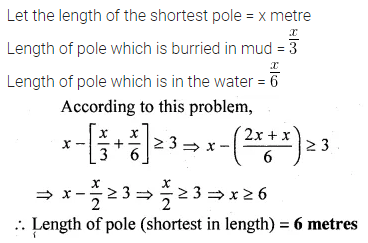# ML Aggarwal Class 10 Solutions for ICSE Maths Chapter 4 Linear Inequations Ex 4

## ML Aggarwal Class 10 Solutions for ICSE Maths Chapter 4 Linear Inequations Ex 4

ML Aggarwal Class 10 Solutions for ICSE Maths Chapter 4 Linear Inequations Ex 4

Question 1.
Solve the inequation 3x – 11 < 3 where x ∈ {1, 2, 3,……, 10}. Also, represent its solution on a number line
Solution: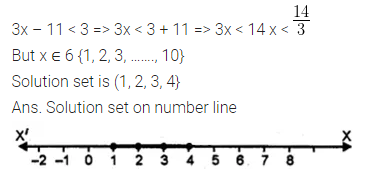Question 2.
Solve 2(x – 3) < 1, x ∈ {1, 2, 3, …. 10}
Solution:Question 3.
$$E=mc^2$$
Solve 5 – 4x > 2 – 3x, x ∈ W. Also represent its solution on the number line.
Solution: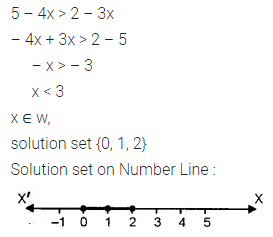Question 4.
List the solution set of 30 – 4 (2x – 1) < 30, given that x is a positive integer.
Solution: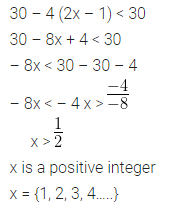Question 5.
Solve : 2 (x – 2) < 3x – 2, x ∈ { – 3, – 2, – 1, 0, 1, 2, 3} .
Solution:Question 6.
If x is a negative integer, find the solution set of $$\\ \frac { 2 }{ 3 }$$+$$\\ \frac { 1 }{ 3 }$$ (x + 1) > 0.
Solution: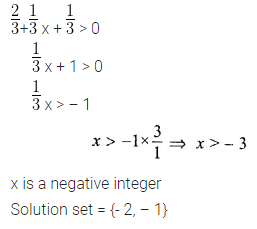Question 7.
Solve: $$\frac { 2x-3 }{ 4 } \ge \frac { 1 }{ 2 }$$, x ∈ {0, 1, 2,…,8}
Solution:Question 8.
Solve x – 3 (2 + x) > 2 (3x – 1), x ∈ { – 3, – 2, – 1, 0, 1, 2, 3}. Also represent its solution on the number line.
Solution: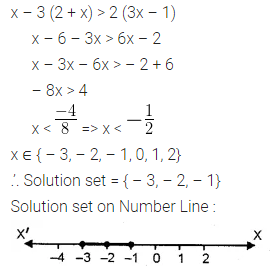Question 9.
Given x ∈ {1, 2, 3, 4, 5, 6, 7, 9} solve x – 3 < 2x – 1.
Solution: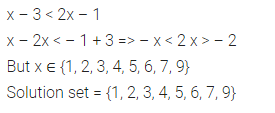Question 10.
Given A = {x : x ∈ I, – 4 ≤ x ≤ 4}, solve 2x – 3 < 3 where x has the domain A Graph the solution set on the number line.
Solution: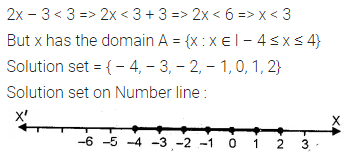Question 11.
List the solution set of the inequation
$$\\ \frac { 1 }{ 2 }$$ + 8x > 5x $$– \frac { 3 }{ 2 }$$, x ∈ Z
Solution: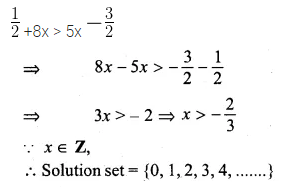Question 12.
List the solution set of $$\\ \frac { 11-2x }{ 5 }$$ ≥ $$\\ \frac { 9-3x }{ 8 }$$ + $$\\ \frac { 3 }{ 4 }$$, x ∈ N
Solution: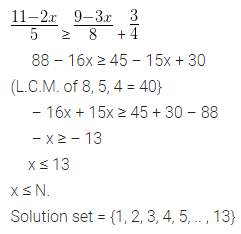Question 13.
Find the values of x, which satisfy the inequation : $$-2\le \frac { 1 }{ 2 } -\frac { 2x }{ 3 } \le 1\frac { 5 }{ 6 }$$, x ∈ N. Graph the solution set on the number line. (2001)
Solution: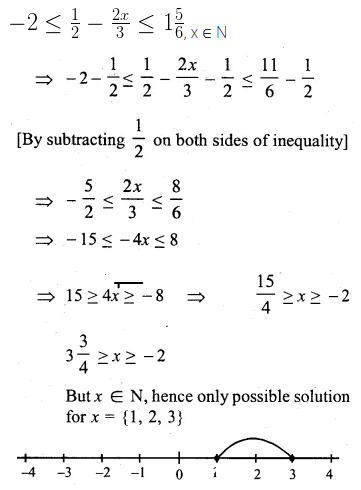Question 14.
If x ∈ W, find the solution set of
$$\frac { 3 }{ 5 } x-\frac { 2x-1 }{ 3 } >1$$
Also graph the solution set on the number line, if possible.
Solution: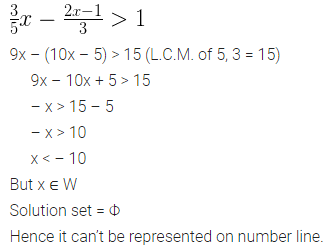Question 15.
Solve:
(i)$$\frac { x }{ 2 } +5\le \frac { x }{ 3 } +6$$ where x is a positive odd integer.
(ii)$$\frac { 2x+3 }{ 3 } \ge \frac { 3x-1 }{ 4 }$$ where x is positive even integer.
Solution:Question 16.
Given that x ∈ I, solve the inequation and graph the solution on the number line:
$$3\ge \frac { x-4 }{ 2 } +\frac { x }{ 3 } \ge 2$$ (2004)
Solution:Question 17.
Given x ∈ {1, 2, 3, 4, 5, 6, 7, 9}, find the values of x for which -3 < 2x – 1 < x + 4.
Solution: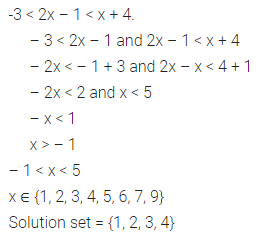Question 18.
Solve : 1 ≥ 15 – 7x > 2x – 27, x ∈ N
Solution:Question 19.
If x ∈ Z, solve 2 + 4x < 2x – 5 ≤ 3x. Also represent its solution on the number line.
Solution: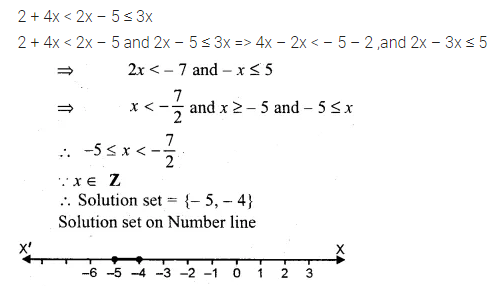Question 20.
Solve the inequation = 12 + $$1 \frac { 5 }{ 6 } x$$ ≤ 5 + 3x, x ∈ R. Represent the solution on a number line. (1999)
Solution: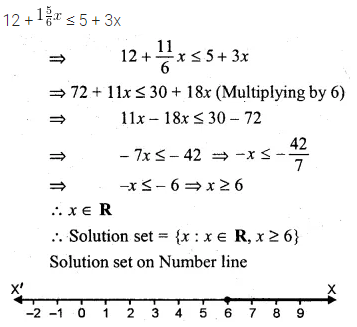Question 21.
Solve: $$\\ \frac { 4x-10 }{ 3 }$$≤$$\\ \frac { 5x-7 }{ 2 }$$ x ∈ R and represent the solution set on the number line.
Solution:Question 22.
Solve $$\frac { 3x }{ 5 } -\frac { 2x-1 }{ 3 }$$ > 1, x ∈ R and represent the solution set on the number line.
Solution:Question 23.
Solve the inequation – 3 ≤ 3 – 2x < 9, x ∈ R. Represent your solution on a number line. (2000)
Solution:Question 24.
Solve 2 ≤ 2x – 3 ≤ 5, x ∈ R and mark it on a number line. (2003)
Solution:Question 25.
Given that x ∈ R, solve the following inequation and graph the solution on the number line: -1 ≤ 3 + 4x < 23. (2006)
Solution:Question 26.
Solve tlie following inequation and graph the solution on the number line. (2007)
$$-2\frac { 2 }{ 3 } \le x+\frac { 1 }{ 3 } <3+\frac { 1 }{ 3 }$$ , x ∈ R
Solution:Question 27.
Solve the following inequation and represent the solution set on the number line:
$$-3<-\frac { 1 }{ 2 } -\frac { 2x }{ 3 } \le \frac { 5 }{ 6 } ,x\in R$$
Solution:Question 28.
Solve $$\frac { 2x+1 }{ 2 } +2(3-x)\ge 7,x\in R$$. Also graph the solution set on the number line
Solution: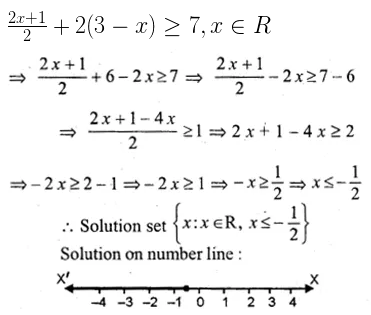Question 29.
Solving the following inequation, write the solution set and represent it on the number line. – 3(x – 7) ≥ 15 – 7x > $$\\ \frac { x+1 }{ 3 }$$, n ∈ R
Solution:Question 30.
Solve the inequation :
$$-2\frac { 1 }{ 2 } +2x\le \frac { 4x }{ 3 } \le \frac { 4 }{ 3 } +2x,\quad x\in W$$. Graph the solution set on the number line.
Solution: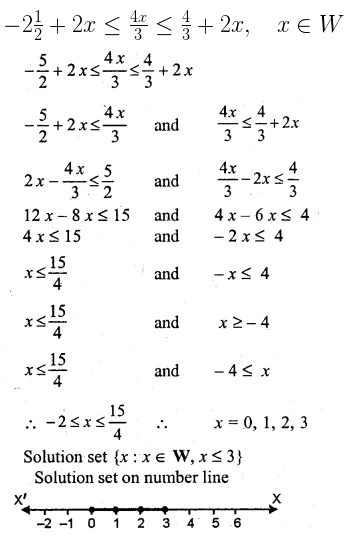Question 31.
Solve the inequation 2x – 5 ≤ 5x + 4 < 11, where x ∈ I. Also represent the solution set on the number line. (2011)
Solution: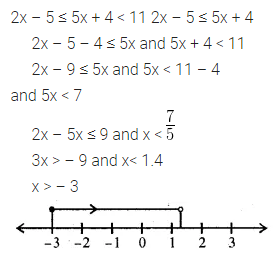Question 32.
If x ∈ I, A is the solution set of 2 (x – 1) < 3 x – 1 and B is the solution set of 4x – 3 ≤ 8 + x, find A ∩ B.
Solution:Question 33.
If P is the solution set of -3x + 4 < 2x – 3, x ∈ N and Q is the solution set of 4x – 5 < 12, x ∈ W, find
(i) P ∩ Q
(ii) Q – P.
Solution:Question 34.
A = {x : 11x – 5 > 7x + 3, x ∈ R} and B = {x : 18x – 9 ≥ 15 + 12x, x ∈ R}
Find the range of set A ∩ B and represent it on a number line
Solution:Question 35.
Given: P {x : 5 < 2x – 1 ≤ 11, x ∈ R)
Q {x : – 1 ≤ 3 + 4x < 23, x ∈ I) where
R = (real numbers), I = (integers)
Represent P and Q on number line. Write down the elements of P ∩ Q. (1996)
Solution: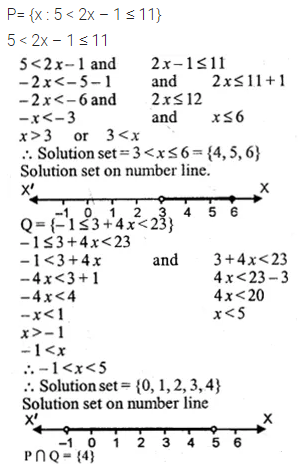Question 36.
If x ∈ I, find the smallest value of x which satisfies the inequation $$2x+\frac { 5 }{ 2 } >\frac { 5x }{ 3 } +2$$
Solution:Question 37.
Given 20 – 5 x < 5 (x + 8), find the smallest value of x, when
(i) x ∈ I
(ii) x ∈ W
(iii) x ∈ N.
Solution: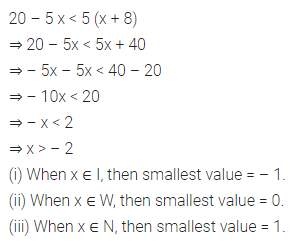Question 38.
Solve the following inequation and represent the solution set on the number line:
$$4x-19<\frac { 3x }{ 5 } -2\le -\frac { 2 }{ 5 } +x,x\in R$$
Solution: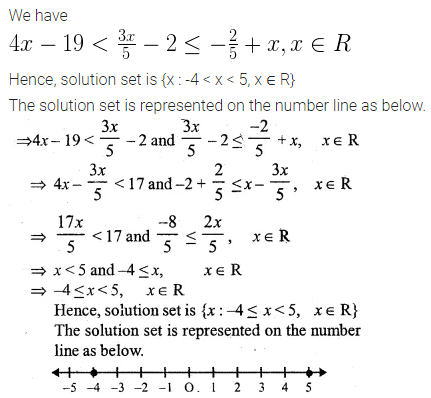Question 39.
Solve the given inequation and graph the solution on the number line:
2y – 3 < y + 1 ≤ 4y + 7; y ∈ R.
Solution: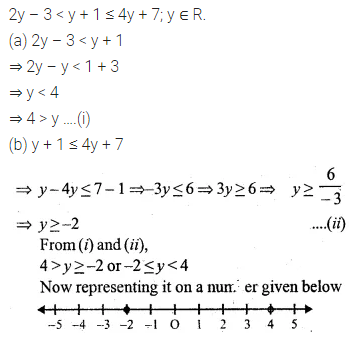Question 40.
Solve the inequation and represent the solution set on the number line.
$$-3+x\le \frac { 8x }{ 3 } +2\le \frac { 14 }{ 3 } +2x,Where\quad x\in I$$
Solution: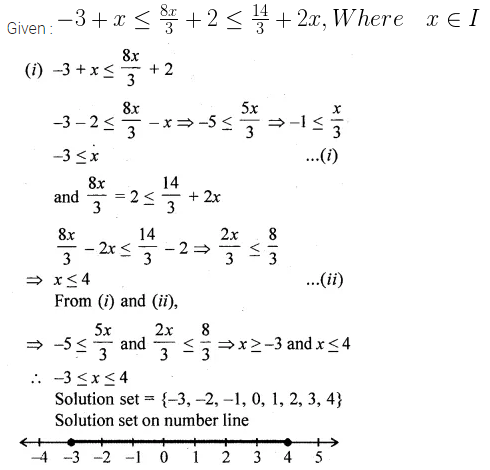Question 41.
Find the greatest integer which is such that if 7 is added to its double, the resulting number becomes greater than three times the integer.
Solution:Question 42.
One-third of a bamboo pole is buried in mud, one-sixth of it is in water and the part above the water is greater than or equal to 3 metres. Find the length of the shortest pole.
Solution: